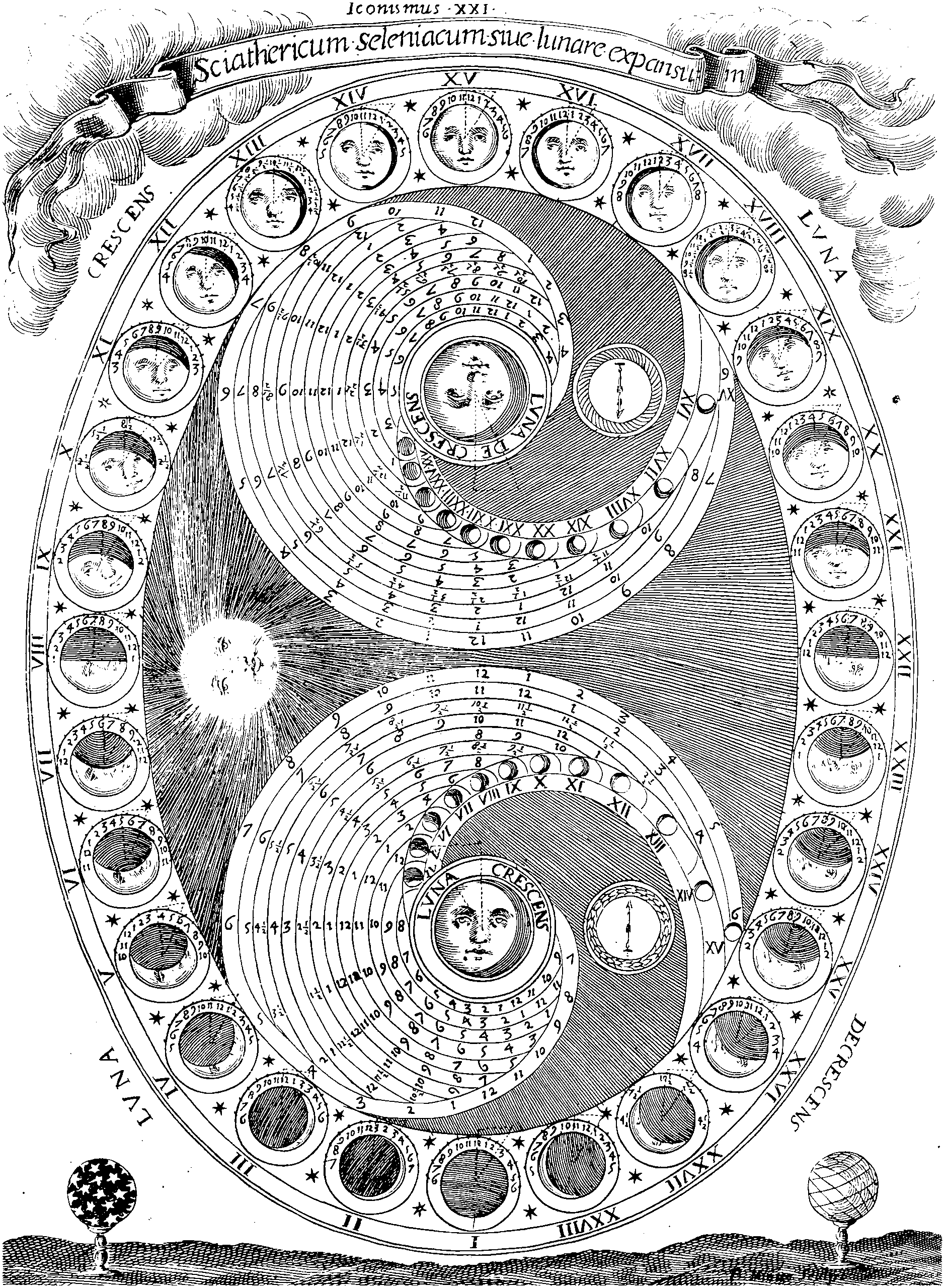# Kernel approximationA page where I document what I don’t know about kernel approximation. A page about what I do know would be empty.

What I mean is: approximating implicit Mercer kernel feature maps with explicit features; Equivalently, approximating the Gram matrix, which is also related to mixture model inference and clustering.

I’m especially interested in how it might be done using random linear algebra via random embeddings such as random projections.

Also I guess we need to know trade-offs of computational time/space cost in approximation bounds, so that we can decide when to bother. When is it enough to reduce computational cost merely with support vectors, or to evaluate the kernels efficiently using, e.g. the Fast Gauss Transform and related methods, rather than coming up with alternative kernel bases? (e.g. you don’t want to do the fiddly coding for the Faust Gauss transform)

Not the related but distinct (terminological collision) of approximating functions from convolution kernels. In kernel approximation we look for approximations, in some sense, to the component kernels themselves.

A good introduction to what is going on is

## Do you need to even calculate the Gram matrix?

Francis R. Bach and Jordan (2002) does a kernel ICA without calculating the whole Gram matrix (!). Does that fit in here?

## Stationary kernels

I am mostly interested in translation-invariant (i.e. stationary, or homogeneous) kernels, so assume I’m talking about those unless I say otherwise.

Short introduction at scikit-learn kernel approximation page.

The approximations might be random projection, or random sampling based, e.g. the Nyström method, which is reportedly effectively Monte Carlo integration, although I understand there is an optimisation step too?

I need to work out the difference between random Fourier features, random kitchen sinks, Nyström methods and whatever Smola et al call their special case Gaussian approximation. I think Random Fourier features are the same as random kitchen sinks, Rahimi and Recht (2007) and Nyström is different . When we can exploit (data- or kernel-) structure to do better? (say, ) Quasi Monte Carlo can improve on random Monte Carlo? (update: someone already had that idea: ) Or better matrix factorisations?

Vempati et al. (2010):

Recently, Maji and Berg and Vedaldi and Zisserman proposed explicit feature maps to approximate the additive kernels (intersection, $$\chi^2$$, etc.) by linear ones, thus enabling the use of fast machine learning technique in a non-linear context. An analogous technique was proposed by Rahimi and Recht for the translation invariant RBF kernels. In this paper, we complete the construction and combine the two techniques to obtain explicit feature maps for the generalized RBF kernels.

## Using bases

See GP basis methods.

## Inner product kernels

See e.g. Koltchinskii and Giné (2000). These have some elegant relations to low-dimensional random projections. How well can we approximate NN kernels?

## LecturesInkedIcon, on inversions in Hilbert space, after Charles Stross

## Connections

MDS and Kernel PCA and mixture models are all related in a way I should try to understand when I have a moment.

For the connection with kernel PCA, see Schölkopf et al. (1998) and C. K. I. Williams (2001) for metric multidimensional scaling.

Altschuler et al. (2019) use projection tricks to calculate Sinkhorn divergence.

### No comments yet. Why not leave one?

GitHub-flavored Markdown & a sane subset of HTML is supported.